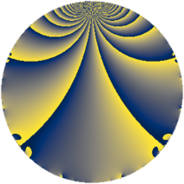# Properties

 Label 1183.2.aLevel $1183$ Weight $2$ Character orbit 1183.a Rep. character $\chi_{1183}(1,\cdot)$ Character field $\Q$ Dimension $77$ Newform subspaces $18$ Sturm bound $242$ Trace bound $6$

# Related objects

## Defining parameters

 Level: $$N$$ $$=$$ $$1183 = 7 \cdot 13^{2}$$ Weight: $$k$$ $$=$$ $$2$$ Character orbit: $$[\chi]$$ $$=$$ 1183.a (trivial) Character field: $$\Q$$ Newform subspaces: $$18$$ Sturm bound: $$242$$ Trace bound: $$6$$ Distinguishing $$T_p$$: $$2$$, $$11$$

## Dimensions

The following table gives the dimensions of various subspaces of $$M_{2}(\Gamma_0(1183))$$.

Total New Old
Modular forms 134 77 57
Cusp forms 107 77 30
Eisenstein series 27 0 27

The following table gives the dimensions of the cuspidal new subspaces with specified eigenvalues for the Atkin-Lehner operators and the Fricke involution.

$$7$$$$13$$FrickeDim.
$$+$$$$+$$$$+$$$$17$$
$$+$$$$-$$$$-$$$$21$$
$$-$$$$+$$$$-$$$$24$$
$$-$$$$-$$$$+$$$$15$$
Plus space$$+$$$$32$$
Minus space$$-$$$$45$$

## Trace form

 $$77q + q^{2} + 4q^{3} + 81q^{4} - 2q^{5} + q^{7} - 3q^{8} + 81q^{9} + O(q^{10})$$ $$77q + q^{2} + 4q^{3} + 81q^{4} - 2q^{5} + q^{7} - 3q^{8} + 81q^{9} - 10q^{10} + 4q^{11} - q^{14} + 4q^{15} + 85q^{16} - 2q^{17} + 9q^{18} + 12q^{19} + 2q^{20} - 4q^{22} - 12q^{23} + 4q^{24} + 67q^{25} + 4q^{27} + 7q^{28} - 18q^{29} - 16q^{30} + 4q^{31} - 15q^{32} + 4q^{33} - 2q^{34} - 6q^{35} + 93q^{36} + 6q^{37} + 8q^{38} - 14q^{40} - 2q^{41} + 8q^{42} - 16q^{43} - 22q^{45} + 16q^{46} - 8q^{47} - 28q^{48} + 77q^{49} - q^{50} - 8q^{51} + 14q^{53} - 48q^{54} - 28q^{55} + 3q^{56} - 30q^{58} - 16q^{59} + 20q^{60} + 14q^{61} - 4q^{62} + 5q^{63} + 73q^{64} - 36q^{66} + 16q^{67} - 54q^{68} + 4q^{69} - 6q^{70} + 32q^{71} + q^{72} + 22q^{73} - 54q^{74} + 32q^{75} - 16q^{76} - 4q^{77} - 12q^{79} + 38q^{80} + 77q^{81} - 42q^{82} - 24q^{83} - 16q^{84} + 8q^{85} + 24q^{86} + 8q^{87} - 84q^{88} - 26q^{89} + 2q^{90} - 8q^{92} + 20q^{93} - 20q^{94} - 16q^{95} + 4q^{96} + 6q^{97} + q^{98} - 32q^{99} + O(q^{100})$$

## Decomposition of $$S_{2}^{\mathrm{new}}(\Gamma_0(1183))$$ into newform subspaces

Label Dim. $$A$$ Field CM Traces A-L signs $q$-expansion
$$a_2$$ $$a_3$$ $$a_5$$ $$a_7$$ 7 13
1183.2.a.a $$1$$ $$9.446$$ $$\Q$$ None $$0$$ $$-2$$ $$3$$ $$-1$$ $$+$$ $$+$$ $$q-2q^{3}-2q^{4}+3q^{5}-q^{7}+q^{9}+4q^{12}+\cdots$$
1183.2.a.b $$1$$ $$9.446$$ $$\Q$$ None $$2$$ $$0$$ $$3$$ $$1$$ $$-$$ $$+$$ $$q+2q^{2}+2q^{4}+3q^{5}+q^{7}-3q^{9}+\cdots$$
1183.2.a.c $$2$$ $$9.446$$ $$\Q(\sqrt{5})$$ None $$-3$$ $$-3$$ $$-3$$ $$-2$$ $$+$$ $$+$$ $$q+(-1-\beta )q^{2}+(-1-\beta )q^{3}+3\beta q^{4}+\cdots$$
1183.2.a.d $$2$$ $$9.446$$ $$\Q(\sqrt{2})$$ None $$0$$ $$0$$ $$-6$$ $$-2$$ $$+$$ $$+$$ $$q+\beta q^{2}+\beta q^{3}+(-3+\beta )q^{5}+2q^{6}+\cdots$$
1183.2.a.e $$2$$ $$9.446$$ $$\Q(\sqrt{3})$$ None $$0$$ $$2$$ $$0$$ $$-2$$ $$+$$ $$+$$ $$q+\beta q^{2}+(1-\beta )q^{3}+q^{4}+\beta q^{5}+(-3+\cdots)q^{6}+\cdots$$
1183.2.a.f $$2$$ $$9.446$$ $$\Q(\sqrt{3})$$ None $$0$$ $$2$$ $$0$$ $$2$$ $$-$$ $$+$$ $$q+\beta q^{2}+(1+\beta )q^{3}+q^{4}+\beta q^{5}+(3+\cdots)q^{6}+\cdots$$
1183.2.a.g $$2$$ $$9.446$$ $$\Q(\sqrt{5})$$ None $$3$$ $$-3$$ $$3$$ $$2$$ $$-$$ $$+$$ $$q+(1+\beta )q^{2}+(-1-\beta )q^{3}+3\beta q^{4}+\cdots$$
1183.2.a.h $$3$$ $$9.446$$ 3.3.148.1 None $$-2$$ $$0$$ $$-3$$ $$3$$ $$-$$ $$-$$ $$q+(-1+\beta _{1})q^{2}+\beta _{2}q^{3}+(1-\beta _{1}+\beta _{2})q^{4}+\cdots$$
1183.2.a.i $$3$$ $$9.446$$ 3.3.316.1 None $$-1$$ $$-2$$ $$-2$$ $$3$$ $$-$$ $$+$$ $$q-\beta _{1}q^{2}+(-1+\beta _{1}-\beta _{2})q^{3}+(1+\beta _{2})q^{4}+\cdots$$
1183.2.a.j $$3$$ $$9.446$$ 3.3.148.1 None $$2$$ $$0$$ $$3$$ $$-3$$ $$+$$ $$-$$ $$q+(1-\beta _{1})q^{2}+\beta _{2}q^{3}+(1-\beta _{1}+\beta _{2})q^{4}+\cdots$$
1183.2.a.k $$4$$ $$9.446$$ 4.4.27004.1 None $$-1$$ $$1$$ $$-7$$ $$-4$$ $$+$$ $$+$$ $$q-\beta _{1}q^{2}-\beta _{3}q^{3}+(1+\beta _{1}+\beta _{2})q^{4}+\cdots$$
1183.2.a.l $$4$$ $$9.446$$ 4.4.27004.1 None $$1$$ $$1$$ $$7$$ $$4$$ $$-$$ $$+$$ $$q+\beta _{1}q^{2}-\beta _{3}q^{3}+(1+\beta _{1}+\beta _{2})q^{4}+\cdots$$
1183.2.a.m $$6$$ $$9.446$$ 6.6.7674048.1 None $$-4$$ $$0$$ $$-6$$ $$6$$ $$-$$ $$-$$ $$q+(-1+\beta _{1})q^{2}-\beta _{4}q^{3}+(1-\beta _{1}+\beta _{2}+\cdots)q^{4}+\cdots$$
1183.2.a.n $$6$$ $$9.446$$ 6.6.1279733.1 None $$-2$$ $$-4$$ $$-2$$ $$6$$ $$-$$ $$-$$ $$q+(-1+\beta _{2}+\beta _{4})q^{2}+(-\beta _{1}+\beta _{3}+\cdots)q^{3}+\cdots$$
1183.2.a.o $$6$$ $$9.446$$ 6.6.1279733.1 None $$2$$ $$-4$$ $$2$$ $$-6$$ $$+$$ $$+$$ $$q+(1-\beta _{2}-\beta _{4})q^{2}+(-\beta _{1}+\beta _{3})q^{3}+\cdots$$
1183.2.a.p $$6$$ $$9.446$$ 6.6.7674048.1 None $$4$$ $$0$$ $$6$$ $$-6$$ $$+$$ $$-$$ $$q+(1-\beta _{1})q^{2}-\beta _{4}q^{3}+(1-\beta _{1}+\beta _{2}+\cdots)q^{4}+\cdots$$
1183.2.a.q $$12$$ $$9.446$$ $$\mathbb{Q}[x]/(x^{12} - \cdots)$$ None $$-3$$ $$8$$ $$4$$ $$-12$$ $$+$$ $$-$$ $$q-\beta _{1}q^{2}+(1+\beta _{6})q^{3}+(2-\beta _{4}+\beta _{5}+\cdots)q^{4}+\cdots$$
1183.2.a.r $$12$$ $$9.446$$ $$\mathbb{Q}[x]/(x^{12} - \cdots)$$ None $$3$$ $$8$$ $$-4$$ $$12$$ $$-$$ $$+$$ $$q+\beta _{1}q^{2}+(1+\beta _{6})q^{3}+(2-\beta _{4}+\beta _{5}+\cdots)q^{4}+\cdots$$

## Decomposition of $$S_{2}^{\mathrm{old}}(\Gamma_0(1183))$$ into lower level spaces

$$S_{2}^{\mathrm{old}}(\Gamma_0(1183)) \cong$$ $$S_{2}^{\mathrm{new}}(\Gamma_0(91))$$$$^{\oplus 2}$$$$\oplus$$$$S_{2}^{\mathrm{new}}(\Gamma_0(169))$$$$^{\oplus 2}$$